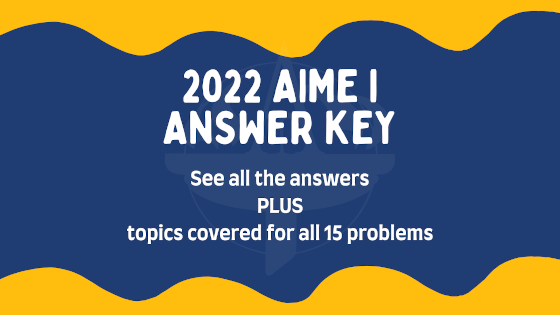# 2022 AIME I Answer Key ReleasedPosted by

Yesterday, select middle school and high school students participated in this year’s AIME I Competition (including some students proctored by Areteem online).

The problems can now be discussed! See below for the answer key for the 2022 AIME I questions as well as the concepts tested on each problem. All answers for the AIME are in the form of 3-digit integers.

1
116
2
227
number theory: bases and digits
3
242
geometry: trapezoids, angle bisectors, and similar triangles
4
834
algebra and number theory: complex numbers, polar form, and modular arithmetic
5
550
algebra and geometry: motion, vectors, equations, and relative speeds
6
228
algebra and combinatorics: sequences, counting, and careful thinking
7
289
algebra and number theory: cases, substitution, and minimums
8
378
geometry: triangles, circles, and tangents
9
247
combinatorics: counting, careful thinking, and permutations
10
756
solid geometry: spheres, cross-sections, and distance formula
11
150
geometry: parallelograms, circles, and power of a point
12
245
combinatorics: sets, counting, sums, and combinatorial identities
13
392
number theory and combinatorics: repeating decimals and counting multiples
14
459
geometry and number theory: triangles, polynomials, and Pythagorean triples
15
33
algebra: geometric interpretations and trigonometric substitutions

Want to practice more AMC and AIME Problems? Check out the Zoom International Math League (ZIML) practice page here for practice resources, including Mock Exams and old Exams.

The AIME I exam is administered by the Mathematical Association of America (MAA). Students are invited to take the AIME based on their scores for the AMC 10 and 12 exams. For general information about the AMC 10 and 12, click here.